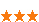您可以捐助，支持我们的公益事业。 1元 10元 50元 认证码：必填求知 文章 文库 Lib 视频 iProcess 课程 认证 咨询 工具 讲座 Modeler Code要资料订阅捐助1048 次浏览     评价： 好 中 差

JavaScript 函数式编程是一个存在了很久的话题，但似乎从 2016 年开始，它变得越来越火热。这可能是因为 ES6 语法对于函数式编程更为友好，也可能是因为诸如 RxJS (ReactiveX) 等函数式框架的流行。`// 数组中每个单词，首字母大写` // 一般写法 const arr = ['apple', 'pen', 'apple-pen']; for(const i in arr){ const c = arr[i]; arr[i] = c.toUpperCase() + arr[i].slice(1); } console.log(arr); // 函数式写法一 function upperFirst(word) { return word.toUpperCase() + word.slice(1); } function wordToUpperCase(arr) { return arr.map(upperFirst); } console.log(wordToUpperCase(['apple', 'pen', 'apple-pen'])); // 函数式写法二 console.log(arr.map(['apple', 'pen', 'apple-pen'], word => word.toUpperCase() + word.slice(1)));

 `// 计算数字之和` // 一般写法 console.log(1 + 2 + 3 - 4) // 函数式写法 function sum(a, b) { return a + b; } function sub(a, b) { return a - b; } console.log(sub(sum(sum(1, 2), 3), 4);

 `// 优化写法 (嗯，你没看错，这就是 lodash 的链式写法)const utils = { chain(a) { this._temp = a; return this; }, sum(b) { this._temp += b; return this; }, sub(b) { this._temp -= b; return this; }, value() { const _temp = this._temp; this._temp = undefined; return _temp; }};` console.log(utils.chain(1).sum(2).sum(3).sub(4).value());

 `// 顺序请求两个接口` // 回调函数 import \$ from 'jquery'; \$.post('a/url/to/target', (rs) => { if(rs){ \$.post('a/url/to/another/target', (rs2) => { if(rs2){ \$.post('a/url/to/third/target'); } }); } }); // Promise import request from 'catta'; // catta 是一个轻量级请求工具，支持 fetch,jsonp,ajax，无依赖 request('a/url/to/target') .then(rs => rs ? \$.post('a/url/to/another/target') : Promise.reject()) .then(rs2 => rs2 ? \$.post('a/url/to/third/target') : Promise.reject());

 `// 创建一个闭包function makeCounter() { let k = 0;` return function() { return ++k; }; } const counter = makeCounter(); console.log(counter()); // 1 console.log(counter()); // 2

makeCounter 这个函数的代码块，在返回的函数中，对局部变量 k ，进行了引用，导致局部变量无法在函数执行结束后，被系统回收掉，从而产生了闭包。而这个闭包的作用就是，“保留住“ 了局部变量，使内层函数调用时，可以重复使用该变量；而不同于全局变量，该变量只能在函数内部被引用。

 `// 简单的缓存工具// 匿名函数创造了一个闭包const cache = (function() { const store = {};` return { get(key) { return store[key]; }, set(key, val) { store[key] = val; } } }()); cache.set('a', 1); cache.get('a'); // 1

map （映射）

map 作为一个高阶函数，他接受一个函数参数作为映射的逻辑

 `// 数组中每一项加一，组成一个新数组` // 一般写法 const arr = [1,2,3]; const rs = []; for(const n of arr){ rs.push(++n); } console.log(rs) // map改写 const arr = [1,2,3]; const rs = arr.map(n => ++n);

 `// 获取目标文件对基础路径的相对路径` // 一般写法 const BASE = '/path/to/base'; const relativePath = path.relative(BASE, '/some/path'); // _.parical 改写 const BASE = '/path/to/base'; const relativeFromBase = _.partial(path.relative, BASE); const relativePath = relativeFromBase('/some/path');

 `// 数组中每个单词大写，做 Base64` // 一般写法 (其中一种) const arr = ['pen', 'apple', 'applypen']; const rs = []; for(const w of arr){ rs.push(btoa(w.toUpperCase())); } console.log(rs); // _.flow 改写 const arr = ['pen', 'apple', 'applypen']; const upperAndBase64 = _.partialRight(_.map, _.flow(_.upperCase, btoa)); console.log(upperAndBase64(arr));

_.flow 将转大写和转 Base64 的函数的能力合并，生成一个新的函数。方便作为参数函数或后续复用。1048 次浏览  评价: 好 中 差订阅捐助

 深度解析：清理烂代码 如何编写出拥抱变化的代码 重构-使代码更简洁优美 团队项目开发"编码规范"系列文章
 重构-改善既有代码的设计 软件重构v2 代码整洁之道 高质量编程规范
 基于HTML5客户端、Web端的应用开发 HTML 5+CSS 开发 嵌入式C高质量编程 C++高级编程

 Android手机开发（一） 理解Javascript 非典型ajax实践 彻底的Ajax javascript 使用Cookies 使用 jQuery 简化 Ajax 开发

 Struts+Spring+Hibernate 基于J2EE的Web 2.0应用开发 J2EE设计模式和性能调优 Java EE 5企业级架构设计 Java单元测试方法与技术 Java编程方法与技术

 某航空公司IT部 JavaScript实践 某电视软件 HTML5和JavaScript 中航信 JavaScript高级应用开发 大庆油田 web界面Ajax开发技术 和利时 使用AJAX进行WEB应用开发